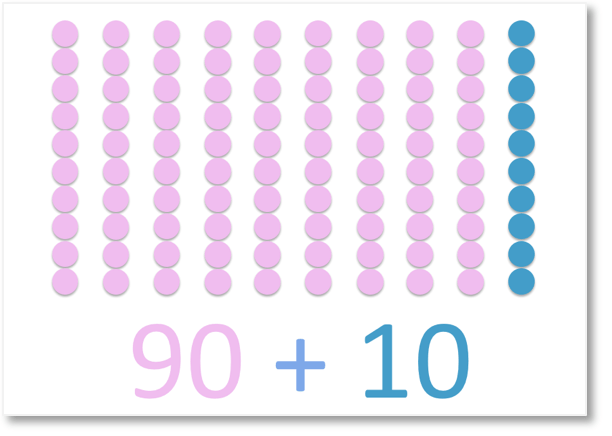# Number Bonds to 20

Number Bonds to 20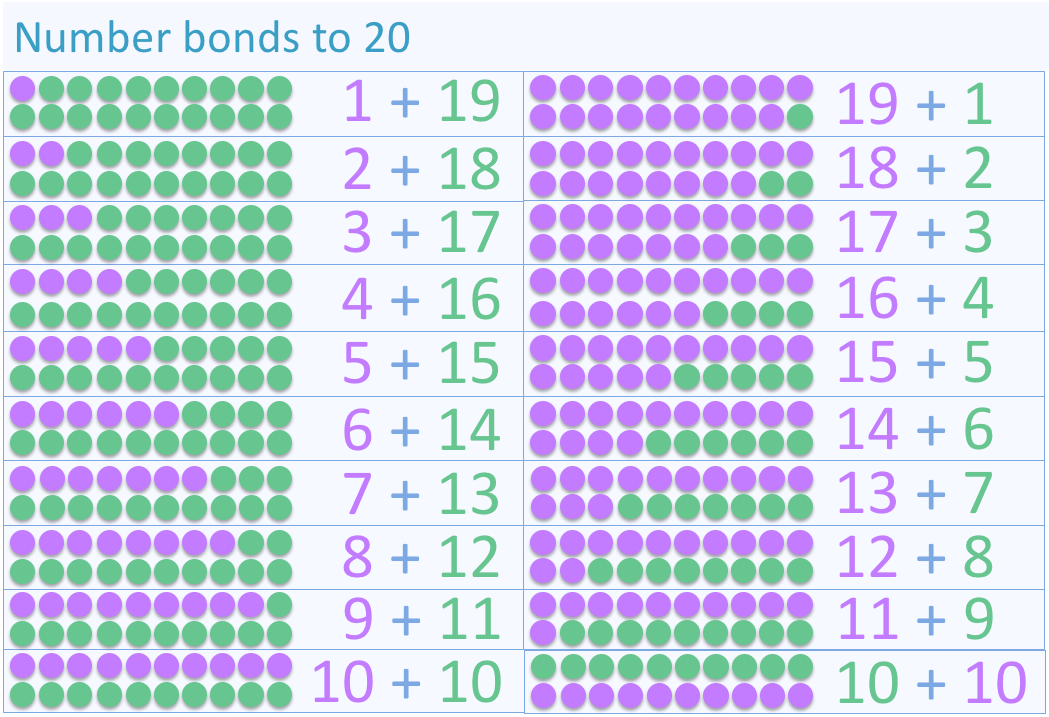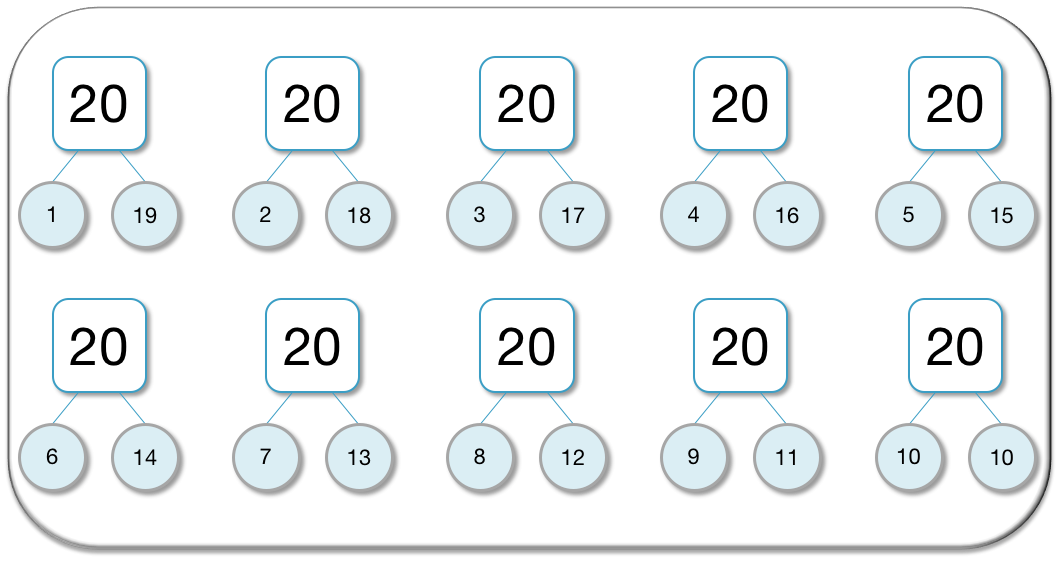• Number bonds to 20 are pairs of numbers that add to make twenty.
• The number bonds to 20 are:
• 1 + 19
• 2 + 18
• 3 + 17
• 4 + 16
• 5 + 15
• 6 + 14
• 7 + 13
• 8 + 12
• 9 + 11
• 10 + 10
• There are 10 different number bonds to 20 to learn.• Counters or cubes can be used as a visual aid to teach number bonds.
• There are ten pairs of number bonds to 20.
• Number bonds to 20 are the number bonds to 10 with an extra ten added to one of the numbers.
• To learn number bonds to 20 we can use patterns.
• 13 + 7 is similar to 17 + 3. We just have the 1 tens digit on the other number.
• The best way to learn the number bonds to 20 is to learn the number bonds to 10 and add 10 to one of the numbers in each pair.

Number Bonds to 20 are pairs of numbers that add to make 20.

There are ten number bonds to 20 to learn.Supporting Lessons#### Number Bonds to 20: Interactive Activity

Number Bonds to 20: Interactive Questions

# What are Number Bonds to 20?

Number bonds to 20 are the pairs of numbers that add together to make twenty.

There are ten number bonds to 20, which are:

• 1 + 19
• 2 + 18
• 3 + 17
• 4 + 16
• 5 + 15
• 6 + 14
• 7 + 13
• 8 + 12
• 9 + 11
• 10 + 10

To learn the number bonds to 20, learn the number bonds to ten and add a 10 to one of the numbers in the pair.

It helps to remember the pairs of digits that make a ten, which are 1 and 9, 2 and 8, 3 and 7, 4 and 6, 5 and 5.

Below is the full display list of the number bonds to 20.We can see that the order of the numbers being added does not matter.

For example: 2 + 18 and 18 + 2 are the same numbers just in a different order. When teaching number bonds to 20, it is important to point this out to children who may not otherwise consciously think of this.

It is easier to memorise the pair of numbers in the sum than memorising the two sums with the numbers in a different order. Instead of remembering both 3 + 17 and 17 + 3, just focus on remembering that 3 and 17 go together.We can show the number bonds to 20 with a visual aid in the form of counters or cubes. This is a useful practical activity for introducing the number bonds and showing the number size and decomposition.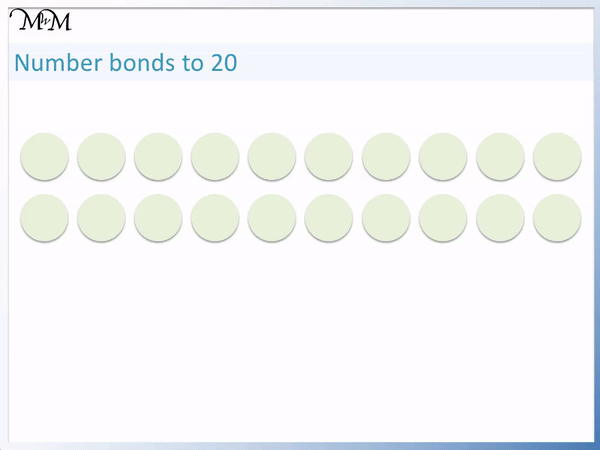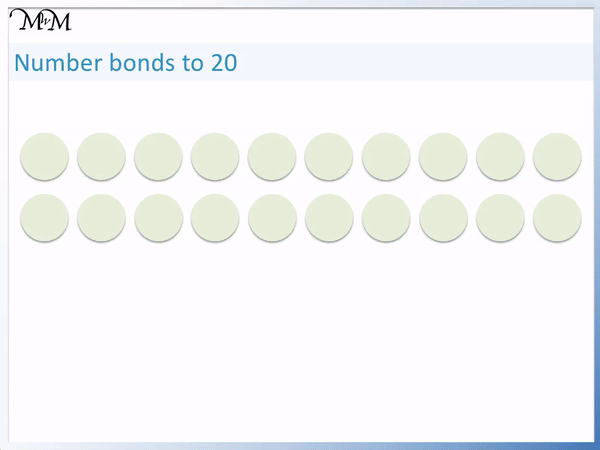Number bonds are typically taught to children from kindergarten to first grade (KS1). When teaching number bonds to 20, it is first important to teach the number bonds to 10. The number bonds to 20 are very similar to the number bonds to 10.

The pairs of numbers that add to make 10 are:

• 1 and 9
• 2 and 8
• 3 and 7
• 4 and 6
• 5 and 5

This is only 5 pairs of numbers. If we remember these, then we can remember the number bonds to 20.

We simply put a ‘1’ tens digit in front of one of the numbers in each pair. This is because the number bonds to 20 are just 10 larger than the number bonds to 10.

Below we can see the number bonds to 20 compared to the number bonds to 10.

Removing the ‘1’ tens digit from the number bonds to 20, we can see that we are left with the number bonds to 10.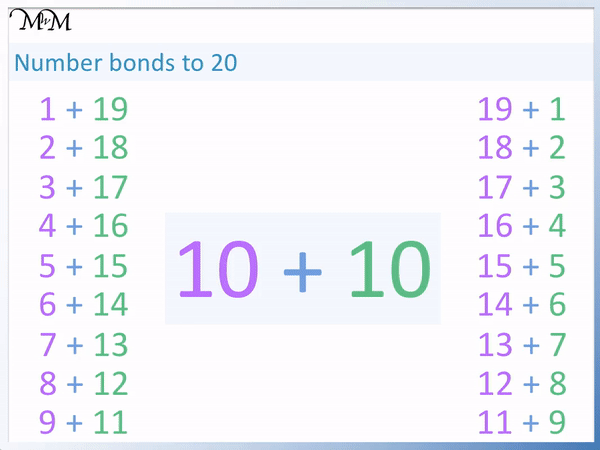Whilst visual aids are the best teaching tool for introducing the idea of number bonds, the best way to actually learn the number bonds to 20 is by first learning the number bonds to 10. We just add a ‘1’ tens digit to one of the numbers in the pair.

When teaching number bonds to 20, it is useful to point out the pattern of increasing and decreasing numbers.

As we increase one number by one, the number that pairs with it decreases by one.

This can help memorise and learn the number bonds to 20.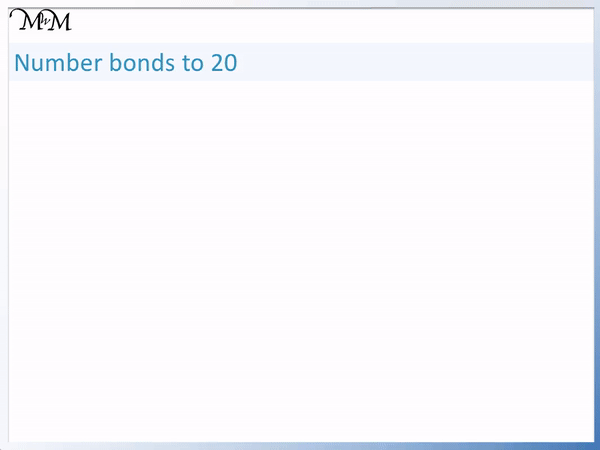For example if we remember that 10 + 10 = 20, then we can increase the first number by 1 and decrease the second number by 1 to get another number bonds to 20. We get 11 + 9. We can continue to increase one number and decrease the other to get 12 + 8 and so on.

Number bonds require practise to learn and memorise. We recommend using our online number bonds to 20 activity to help learn them.

Alternatively, we have part part whole model worksheets. The part part whole teaching model is made up of three circles. The larger number goes in the largest circle and two smaller circles contain the two numbers that add to make this number.

Here are all of the number bonds to 20, shown as part part whole models.The entire list of number bonds to 20 are shown as a display poster below.

If you are teaching number bonds to 20, please feel free to download and print this list.Now try our lesson on Introducing Number Bonds to 100 where we learn the pairs of numbers that add to make 100.